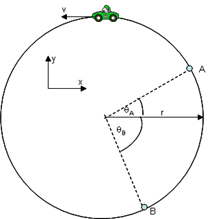# Problem: A car is traveling around a horizontal circular track with radius r = 250 m at a constant speed v = 25 m/s as shown. The angle θA = 35° above the x axis, and the angle θB = 66° below the x axis.1) What is the magnitude of the car’s acceleration?2) What is the x component of the car’s acceleration when it is at point A3) What is the y component of the car’s acceleration when it is at point A4) What is the x component of the car’s acceleration when it is at point B5) What is the y component of the car’s acceleration when it is at point B6) As the car passes point B, the y component of its acceleration is? increasing or constant or decreasing?

###### FREE Expert Solution

Centripetal acceleration:

$\overline{){{\mathbf{a}}}_{{\mathbf{c}}}{\mathbf{=}}\frac{{\mathbf{v}}^{\mathbf{2}}}{\mathbf{r}}{\mathbf{=}}{\mathbf{r}}{{\mathbf{\omega }}}^{{\mathbf{2}}}}$

1.

The magnitude of the car's acceleration is:

$\begin{array}{rcl}{\mathbf{a}}_{\mathbf{c}}& \mathbf{=}& \frac{{\mathbf{v}}^{\mathbf{2}}}{\mathbf{r}}\\ & \mathbf{=}& \frac{{\mathbf{25}}^{\mathbf{2}}}{\mathbf{250}}\end{array}$

90% (415 ratings)###### Problem Details

A car is traveling around a horizontal circular track with radius r = 250 m at a constant speed v = 25 m/s as shown. The angle θA = 35° above the x axis, and the angle θB = 66° below the x axis.1) What is the magnitude of the car’s acceleration?

2) What is the x component of the car’s acceleration when it is at point A

3) What is the y component of the car’s acceleration when it is at point A

4) What is the x component of the car’s acceleration when it is at point B

5) What is the y component of the car’s acceleration when it is at point B

6) As the car passes point B, the y component of its acceleration is? increasing or constant or decreasing?

Frequently Asked Questions

What scientific concept do you need to know in order to solve this problem?

Our tutors have indicated that to solve this problem you will need to apply the Circular Motion concept. You can view video lessons to learn Circular Motion. Or if you need more Circular Motion practice, you can also practice Circular Motion practice problems.